# Marketing

## Quiz 22 :Marketing PlanLooking for Management Homework Help?

## Quiz 22 :Marketing PlanHow does a manufacturer's income statement differ from a retailer's income statement?
Free
Essay

Income statement
Income statement is the detailed record of financial transaction of a firm/company over a given time period. This helps in calculating and determining the net income/loss for the given period of time.
However, most of the items or particulars of income statements of both the retailer and manufacturer remain same but there are differences. Such difference is noticed in the calculation of Cost of goods sold. Procedure of arriving at net income figure is however same i.e., subtracting expenses/losses from income/gain.
Calculation of cost of goods sold is complex in nature in case of manufacturing company. The main reason behind this is the inventories which is maintained at different stages of production by manufacturer namely, raw material inventory, work-in-progress inventory, and finished goods inventory. Costing of such types of inventories is not required to be maintained by the retailer as they get finished goods from manufacturers to be sold to the final consumers.
Calculation of cost of goods sold in manufacturer's income statement considers two elements namely, cost of goods manufactured and cost of goods available for sale. In other words, "purchase" element of retailer's income statement is replaced by "cost of goods manufactured" in manufacturer's income statement.
Other than this, there are manufacturing overheads and related costs in manufacturer income statement whereas retailer's income statement considers expenses such as selling and distribution expenses and other administrative expenses. This is because retailers are not engaged in any kind of manufacturing rather more focused on selling and distributing the final goods.Use the following information to answer parts a, b, and c: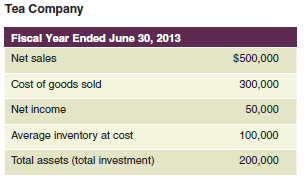a. What is the inventory turnover rate for Tea Company? From what sources will the marketing manager determine the significance of the inventory turnover rate? b. What is the capital turnover ratio? What is the net income ratio? What is the return on investment (ROI)? c. How many dollars of sales did each dollar of investment produce for Tea Company?
Free
Essay

(a)
Calculate the inventory turnover rate
Extracted information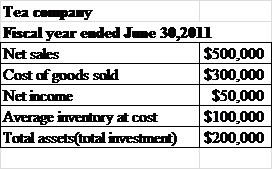Explanation
The current inventory turnover rate can be calculated using the following formula: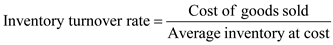Substitute
For cost of goods sold as $300,000 and average inventory at cost as$100,000Thus the inventory turnover rate is 3.
The significance of the inventory turnover ratio can be calculated using the value of the cost of goods sold and average inventory at cost. It should be seen that the cost of goods sold is accurate and is taken on the appropriate costs units.
(b)
Calculate the capital turnover ratio
Capital turnover ratio can be calculated using the following formula: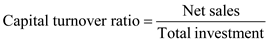Substitute
Net sales as $500,000 and total investment as$200,000Thus the capital turnover ratio is 2.5.
Calculate the net income ratio
Net income ratio can be calculated using the following formula: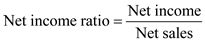Substitute
For net income as $50,000 and net sales as$500,000Thus the net income ratio is 0.1.
Calculate the return on investment
Return on investment can be calculated using the following formula: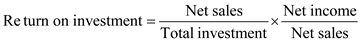Substitute
For net sales as $500,000, net income as$50,000, total investment as $200,000Thus the return on investment is 25%. (c) The amount of investment here is$200,000 and net sales using these assets are $500,000. Thus the amount of dollar earned is$500,000.Product A has a markup percentage on cost of 40 percent. What is the markup percentage on selling price?
Free
Essay

Mark-up can be defined as the difference between the cost and selling price of any product or service.
Mark-up is added to the total cost of manufacturing to earn a profit. It can be expressed both as a percentage or fixed amount of total cost or the selling price.
Mark-up percentage on selling price is calculated by using the formula below: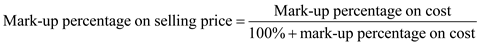In this case,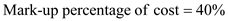On substituting this value in the formula, we getThe mark-up percentage on selling price is 28.57%Product B has a markup percentage on selling price of 30 percent. What is the markup percentage on cost?
EssayProduct C has a cost of $60 and a usual markup percentage of 25 percent on selling price. What price should be placed on this item? Essay Answer:Apex Appliance Company sells 20 units of product Q for$100 each and 10 units for \$80 each. What is the markdown percentage for product Q?